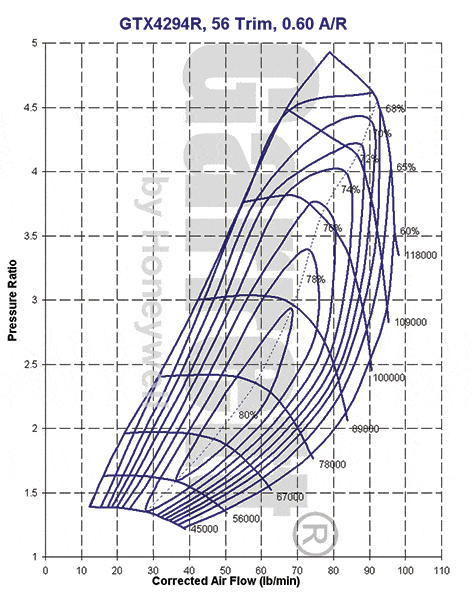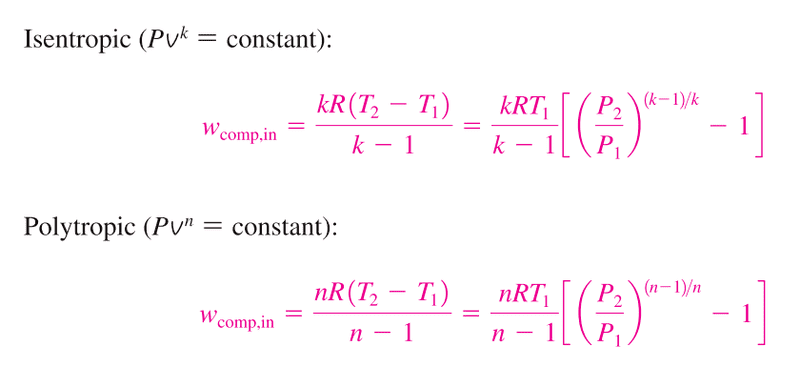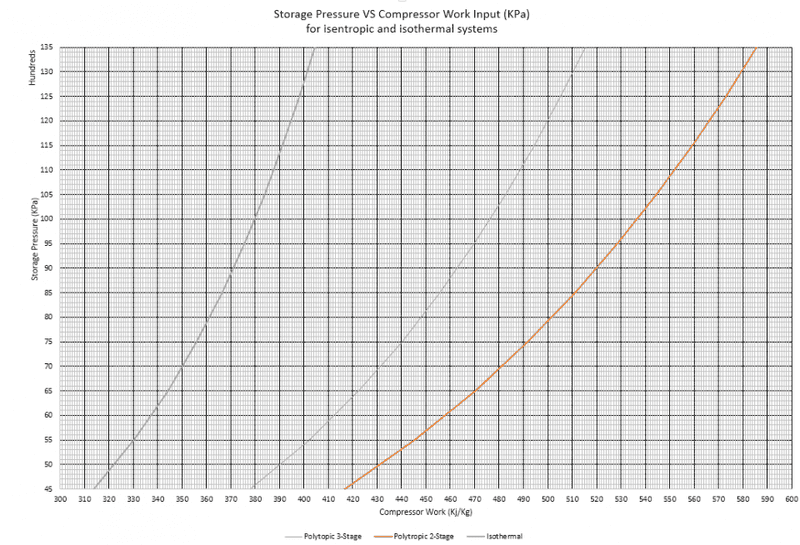# Decoupling Compression and Expansion of a turbine

In summary, the higher the compression pressure the higher the amount of energy per unit mass it will take to compress a given mass of gas.f

Hi,

I just want to double check something: My understanding is that the higher the compression pressure the higher the amount of energy per unit mass it will take to compress a given mass of gas.

So my question is, would a decoupled system where the compression can take place over a longer time period and at lower pressures (case1) be more efficient than one where the compression takes place at the same rate of expansion (case2)?

Here is a bullet point description of my two scenarios:

case 1:
Compressor inlet pressure = 1 bar
Compressor outlet pressure = 50 bar
compression time = t
compression mass rate = x

Turbine inlet pressure = 45 bar
Turbine outlet pressure = 1 bar
Expansion time = t/2
Expansion mass rate = 2x

case 2:
Compressor inlet pressure = 1 bar
Compressor outlet pressure = 100 bar
compression time = t/2
compression mass rate = 2x

Turbine inlet pressure = 45 bar
Turbine outlet pressure = 1 bar
Expansion time = t/2
Expansion mass rate = 2x

I don't really understand your question .

Turbine inlet pressure is compressor outlet pressure minus pressure drop in combustion chamber .

Air flow through compressor is the same as air flow through turbine .

Compressor outlet pressure is designed to be whatever is needed for best efficiency .

Compression is always done in least number of stages possible so as to minimise engine weight and complexity .

I've just posted this on another thread but in case you missed it :

http://er.jsc.nasa.gov/seh/ANASAGUIDETOENGINES.pdf

Please come back if you have specific questions on engine design .

I am actually looking at a design where the compression of the gas and the expansion are not happening at the same time (hence the decoupling). In which case maybe I should have used the term expander rather than turbine.

I hope that this clarifies the question.

Draw me a picture .

Draw me a picture .

I am looking at compressed air energy storage : http://www.intechopen.com/source/html/42268/media/image2_w.jpg

That is a different problem altogether .

You will have to analyse the operating cycle in detail to get any sensible answers .

For a real design project not only the air cycle itself but many practical , economic and environmental factors would have to be considered as well .

My answer would be: In theory, maybe; In practice, no.

Whether coupled or decoupled, a compressor is designed to perform at a certain pressure ratio for a given mass flow rate. For example, here is an efficiency map for the compressor of a turbocharger for a car engine:No matter what you are using this compressor for, it performs best in the center of the middle «island» (80%), where the mass flow rate is about 55 lb/min and the pressure ratio is 2.2. The further you get from this point (in any direction, i.e. higher or lower, pressure ratio or mass flow rate), the worst is your compressor's efficiency. This type of efficiency map can be done for any type of compressor.

So - in theory - if you badly select or design your compressor in case 1 and you switch to case 2, then, yes, you can improve efficiency. But - in practice - no one will badly select or design a compressor on purpose.

Now, if you're talking about designing the correct specific compressor for each case, then they should have similar efficiencies, given the compressors are of the same type.

Thank you for your answer. I am assuming that indeed the "optimal" compressor will be chosen for each scenario, I guess what I want to know is if in case 1 the compressor with an efficiency E will compress at x kJ/kg and the compressor of case 2, also with an efficiency of E, will compress at y kJ/kg, with y > x.

Because when I use the following equations:I end up with graph like these:Which seem to imply that I will expend more energy per unit mass the more I increase my pressure ratio. And no matter if I then multiply that number by an isentropic efficiency, because if the efficiencies are the same then I am still better off compressing longer at lower pressure. Say I want to compress, 100 kg of air, it seems like I'm better of doing it over 2 seconds at 50 kg/s than over 1 second at 100 kg/s.

I apologies if I don't seem to get it but my background is in geosciences.

I' m starting to sense the depth of your cases.

Referring to the OP, the turbine inlet requires 45 bar of pressure. Assuming a Brayton cycle, you need to compress to at least 45 bar. You cannot compress less, by definition.

What happen if you compress to more than 45 bar (say, 100 bar)? On a cycle point of view, nothing. You will have to decompress your stored gas down to 45 bar before letting it go into the combustion chamber, which is giving back to the system the energy you inputted between 45 bar and 100 bar. It is like compressing and decompressing a spring. In practice there will probably be a slight loss.

What would be the point of compressing over 45 bar? The only answer to this is that you could use a smaller volume to store your pre-compressed gas.

So, yes, the lower the pressure, the better it is; as long as you have the minimum required by the cycle. But only because you don't need the extra pressure in the first place, and if you compress the gas to more than what you need, you are doing so only for a reason irrelevant to cycle efficiency.

Great thank you. That is what I suspected. As you say, the reason why you would compress to above turbine inlet pressure is due to other factors hence the geosciences background ;) Basically the container in which I compress my air opposes a resistance to air flow based on the mass flow rates amongst other factors, so if I try and compress my air into it faster than the pressure can dissipate through the medium, then the pressure increases at the entrance of the store and, as you confirmed to me, means my compressor will have to do more work to get the mass to store in the container.